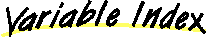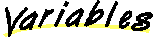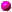`All Packages  Class Hierarchy  This Package  Previous  Next  Index`

# Class logic.BooleanTuple

```java.lang.Object
|
+----logic.BooleanTuple
```

public class BooleanTuple
extends Object
implements BooleanTupleConstants
This class is a wrapper for an array of Booleans. We need a wrapper so that it can be used as a key in a hashtable. It provides a simple implementation of hashCode() for this purpose.

##tuple

##BooleanTuple(Vector)
The constructor accepts a Vector of Conditions -- only 30 are allowed.

##equals(Object)
Two BooleanTuples are equal if they are both nonnull, and their underlying instances of Boolean[] have the same length and have entries that have the same booleanValue()'s.getTuple()hashCode()
The algorithm used here is to treat a sequence of booleans as a binary sequence, with T=1 and F=0, and then treat the binary sequence as an actual binary integer.num(Boolean)setTuple(Boolean[])setTuple(Vector)size()toString()

##tuple
``` private Boolean tuple[]
```

##BooleanTuple
``` public BooleanTuple(Vector tuple) throws NestingTooDeepException
```
The constructor accepts a Vector of Conditions -- only 30 are allowed. It converts the vector to an array and caches it.

##equals
``` public boolean equals(Object ob)
```
Two BooleanTuples are equal if they are both nonnull, and their underlying instances of Boolean[] have the same length and have entries that have the same booleanValue()'s.

Overrides:
equals in class ObjectgetTuple
``` public Boolean[] getTuple()
```hashCode
``` public int hashCode()
```
The algorithm used here is to treat a sequence of booleans as a binary sequence, with T=1 and F=0, and then treat the binary sequence as an actual binary integer. The algorithm simply computes the base 10 value of this binary number and returns it. An inherent limitation is that the largest allowed int is 2^31 -1, so that we can't rely on the algorithm for tuples of length > 30.

Overrides:
hashCode in class Objectnum
``` private int num(Boolean b)
```setTuple
``` public void setTuple(Boolean arr[])
```
Parameters:
v - java.util.VectorsetTuple
``` public void setTuple(Vector v)
```
Parameters:
v - java.util.Vectorsize
``` public int size()
```toString
``` public String toString()
```
Overrides:
toString in class Object

`All Packages  Class Hierarchy  This Package  Previous  Next  Index`